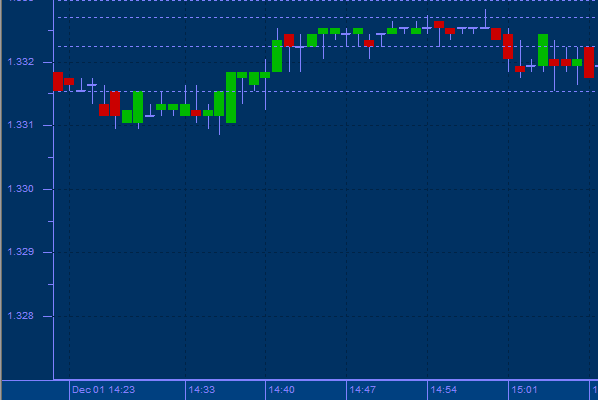top of page## Fibonacci Retracements

Fibonacci numbers are used in calculating Retracements patterns.

For e.g. Many securities, after making long sustained moves in one direction, will eventually retrace a portion of the move before continuing on to extend it. The most popular Retracements amount used is 50%. Meaning, if on its latest move, a stock went from 50 to 100 and then started backing off, a 50% Retracements would bring it to \$75 before it turns around and continues its upward march. In addition to the 50% level, technicians also look at the 38.2% and 61.8% Retracements.

Originally discovered by Pingala in India in 450 B.C., the 12th century mathematician, Leonardo Fibonacci re-discovered this simple numerical series that is the foundation for an incredible mathematical relationship.

Starting with 0 and 1, each new number in the series is simply the sum of the two before it.
0, 1, 1, 2, 3, 5, 8, 13, 21, 34, 55, 89, 144, 233 . . .

Adding the first two numbers to arrive at the third derives the Fibonacci number sequence. The ratio of any number to the next number is 61.8 percent, which is frequently characterized as a major Fibonacci retracement number. The inverse of 61.8 percent is 38.2 percent, also characterized as a major Fibonacci retracement number. Also the ratio of any number in the series to the previous number approaches 1.618 (called ‘Phi’), which some times called the ‘most beautiful number’ by mathematicians since this ratio is widely observed even in seemingly random and unrelated natural phenomenon.

Fibonacci Retracements are based on a trend line drawn between a significant trough and peak. If the trend is rising, the Retracements lines will descend from 100% to 0% .If the trend line is falling, the Retracements lines will ascend from 0% to 100% .Horizontal lines are drawn at the common Fibonacci levels of 38%, 50%, & 62% .As the price retraces, support and resistance often occur at or near the Fibonacci Retracements levels.

Usage:

Markets trend up and down, pause to retrace (consolidate, correct) then continue onward. These Retracements often reclaim constant percentages of the original trend’s move and can be predicted with good accuracy by the Fibonacci sequence. Retracements help anticipate support and resistance levels along with price targets.bottom of page